# Non-hermitian matrices for graph clustering?

GDR ISIS, Mars 2022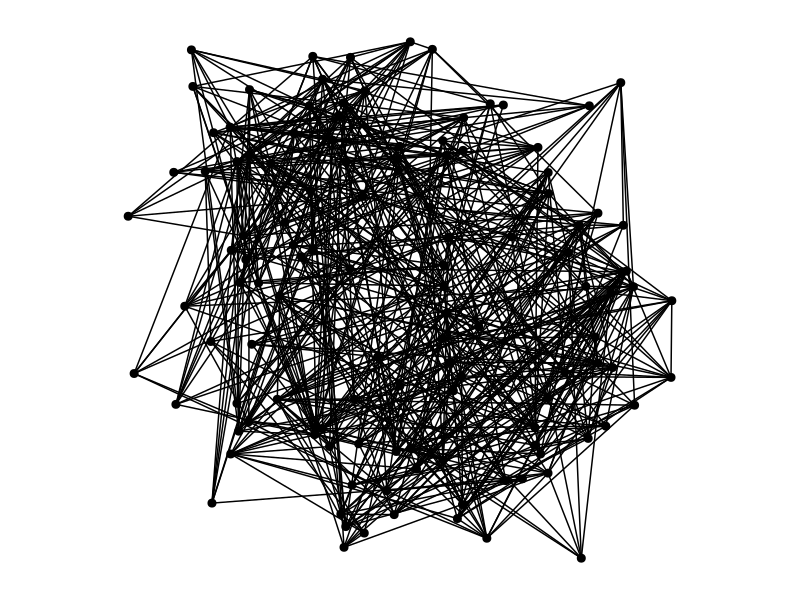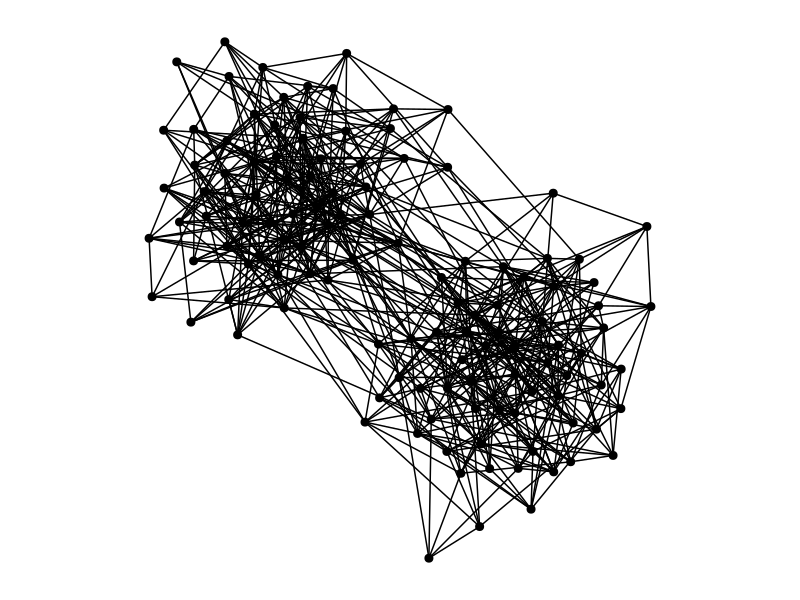# Clustering algorithms in a nutshell

Step Spectral algorithms DL
1 network representation laplacian, adjacency, non-backtracking, Bethe-Hessian… tensorized representations of the graph
2 low-dim node embedding eigenvectors, singular vectors equivariant GNNs
3 clustering on the embedding K-means, EM linear classifier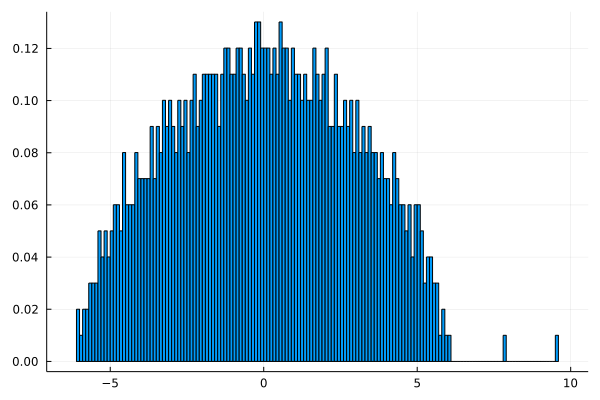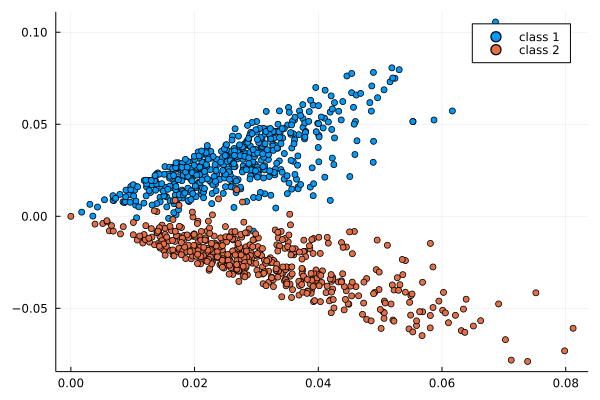lap.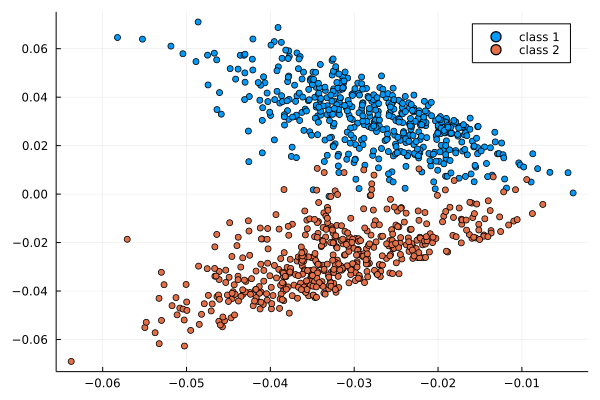asy.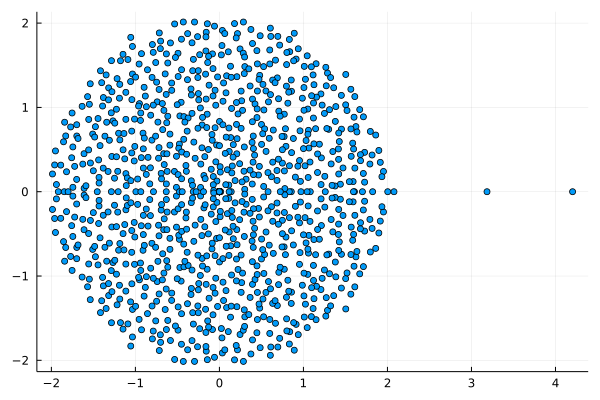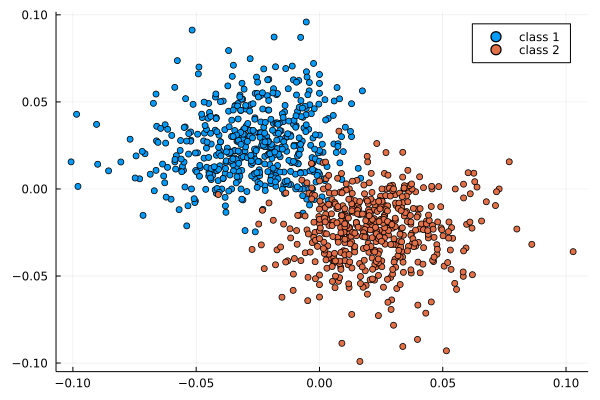# First challenge: sparsity

A graph is sparse when n_\mathrm{edges} \approx n_\mathrm{nodes}.

• classical spectral clustering works when graphs are not too sparse

• classical spectral clustering doesn’t work when graphs are sparse

Potential reasons

Eigenvalues of normal matrices (including SVD) are aligned with high-degrees.

Sparse graphs often have very heterogeneous degrees.

# Second challenge: directedness

• many (most?) real-world networks are directed

• most clustering methods represent digraphs with normal matrices (including SVD-based methods)

# Takeaway messages: Asymmetry helps

1. non-Hermitian matrices have eigenvalues, too

2. non-Hermitian matrices should not frighten you

[Chen, Cheng, Fan, 18] [Bordenave, C, Nadakuditi, 20] [C, Stephan, 21]

# A model of directed inhomogeneous random graphs

Parameters: size n super large, connectivity matrix P=(P_{i,j})

A_{i,j} = edge from node i to node j

A_{i,j} = \mathrm{Bernoulli}(P_{i,j}) ~~~~~~~~~~~~~~~ (\mathrm{independent})

• Sparsity \iff \Vert P \Vert_{\infty} = O(1/n)

• \mathbf{E}[A] = P

• If P has a block-structure as in a SBM, this structure is visible in the eigenvectors

• \lambda_i = eigenvalues of P with left/right eigenvectors u_i, v_i.

• \mu_i = eigenvalues of A with left/right eigenvectors \varphi_i, \psi_i (random).

Theorem (C, Stephan, 2021+)

t = \sqrt{\Vert P \Vert} ~~~~~~~~~~~~ \text{(the crucial threshold)}

• if |\lambda_i| > t then:

\mu_i = \lambda_i + o(1) ~~~~~ \text{and} ~~~~~|\langle u_i, \varphi_i\rangle| \to \mathrm{something}_i

• other eigenvalues of A are smaller than t + o(1).

Usage: estimate u_i from \varphi_i, then find the block-structure

# Example

Here \mathbf{E}[A] has 3 eigenvalues 1,2,3 and t=\sqrt{\Vert P \Vert} \approx 1,37.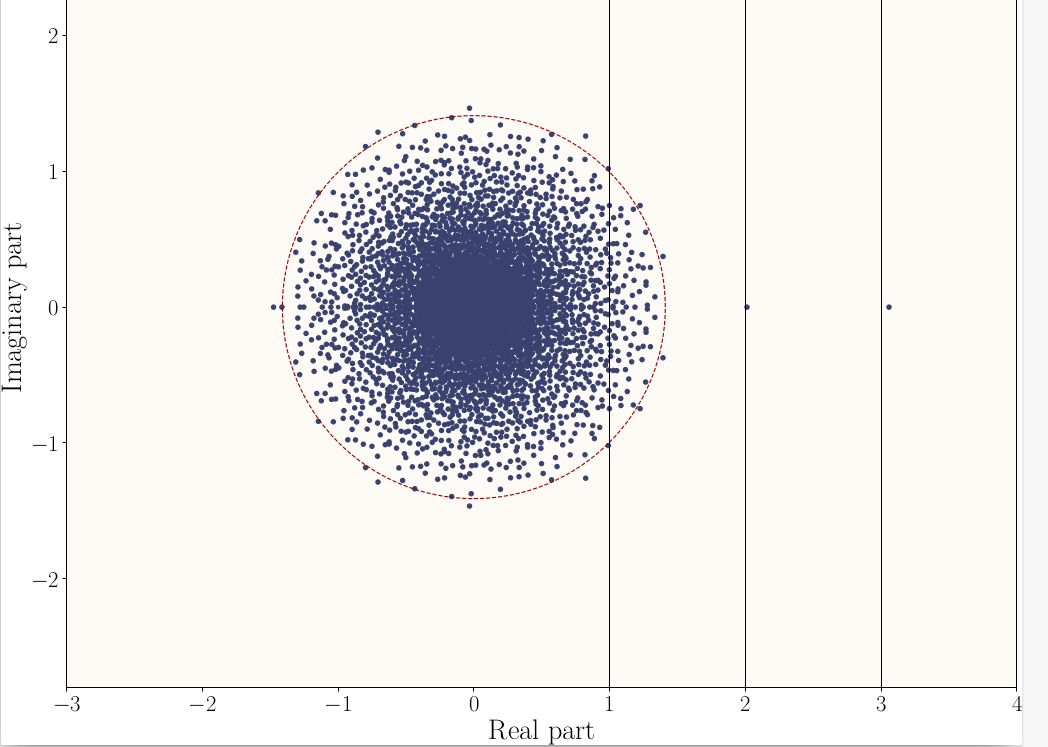# SBM with a block-wise path structure:

P = \frac{d}{n}\begin{bmatrix}1 & 1- \eta & 0 \\ \eta & 1 & 1-\eta & 0 \\ 0 & \eta & 1 & 1 - \eta & \ddots \\ &&&&&&&\\ & & \ddots & \ddots & \ddots \\ \\ \end{bmatrix}

Eigenvalues of P and threshold t=\sqrt{\Vert P \Vert}:

explicit in terms of k (number of blocks), d (density) and \eta (asymmetry)

Green: eig. of an ad hoc skew-Hermitian matrix ([Laenen & Sun 20]) .

d=2 ~~~~~~~~~~~~~~~~~~~~~~~~~ d=5 ~~~~~~~~~~~~~~~~~~~~~~~~~ d=10

# Question: best graph representations for GNNs?

• [Dasoulas, Lutzeyer, Vazirgiannis 21] parametrize their graph matrix representation using a few basic building blocks:
\alpha_1 D^{\gamma_1} + \alpha_2 D^{\gamma_2}A D^{\gamma_3} + \alpha_3 I

They optimize this in \alpha_i, \gamma_j for a fixed GNN architecture.

What happens if you allow truly non-normal representations,

even if the graph is undirected?

and C. Bordenave, L. Massoulié, L. Stephan, L. Dall’Amico…